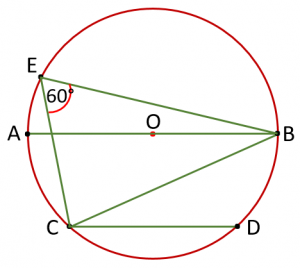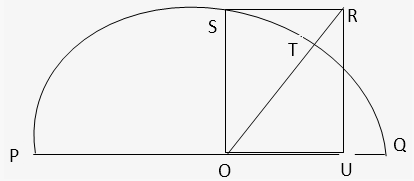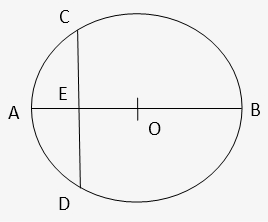Friday, May 7, 2021
Home > geometry > SSC CGL Geometry Circle Set 4

# SSC CGL Geometry Circle Set 4

SSC CGL CHSL Geometry
Tangent to Circle Questions Sets
Set 1Set 2Set 3Set 4

SSC CGL Geometry Circle Set 4 : Ques No 1

If a chord of a circle is equal to its radius, the  angle subtended by the chord at the minor arc of the circle is

Options:

A. 60 degrees

B. 150 degrees

C. 120 degrees

D. 75 degrees

Solution:

SSC CGL Geometry Circle Set 4 : Ques No 2

In a cyclic quadrilateral ABCD, AB and DC when extended meet at point P. if PA = 8 cm, PB = 6 cm and PC = 4 cm, the length of PD in cm is

Options:

A. 10 cm

B. 12 cm

C. 6 cm

D. 8 cm

Solution:

SSC CGL Geometry Circle Set 4 : Ques No 3

In the figure below, chord CD is parallel to diameter AB of a circle with centre at O. If ∠CEB = 65 degrees, then ∠BCD isOptions:

A. 25 degrees

B. 35 degrees

C. 55 degrees

D. 45 degrees

Solution:

SSC CGL Geometry Circle Set 4 : Ques No 4

If ABCD is a cyclic quadrilateral and AD is its diameter. If ∠DAC = 55 degrees, then the value of ∠ABC is

Options:

A. 35 degrees

B. 55 degrees

C. 125 degrees

D. 145 degrees

Solution:

SSC CGL Geometry Circle Set 4 : Ques No 5

The point of intersection of the diagonals AC and BD of the cyclic quadrilateral ABCD is P. If ∠APB = 64 degrees and ∠CBD = 28 degrees, then the value of ∠ADB is

Options:

A. 36 degrees

B. 28 degrees

C. 32 degrees

D. 56 degrees

Solution:

SSC CGL Geometry Circle Set 4 : Ques No 6

A square is inscribed in a quarter circle in such a manner that two of its adjacent vertices lie on two radii at an equal distance from the centre, while the other two vertices lie on the circular arc. If the square has sides of length x, then the radius of the circle is

Options:

A. √5 x/√2

B. √2x

C. 16x/(pi + 4)

D. 2x/√pi

Solution:

SSC CGL Geometry Circle Set 4 : Ques No 7

The wheel of scooter has diameter 140 cm. How many revolutions per minute must the wheel make so that the speed of the scooter is kept at 132 km per hour?

Options:

A. 1100

B. 500

C. 250

D. 1000

SSC CGL Geometry Circle Set 4 : Ques No 8

Two circles with same center are drawn with O as the centre as shown is the figure given below. The ratio of the area of the annular ring bounded by these two circles and the quadrilateral EBCH is 3:2. Find the ratio of the radius of the smaller circle to the radius of the larger circleOptions:

A. 1:4

B. 2:7

C. 1:6

D. 1:7

SSC CGL Geometry Circle Set 4 : Ques No 9

A semicircle having centre at O and radius equal to 4 is drawn with PQ as the diameter as shown is the figure given below. OSRU is a rectangle such that the ratio of area of the semicircle to the area of the rectangle is 2π: 3 or cuts the semicircle at T. Find the length of line segment TQ.Options:

A. (8/5)√5

B. (5/3)√5

C. (17/9)√5

D. (9/2)√5

SSC CGL Geometry Circle Set 4 : Ques No 10

In the circle shown below, AB is a diameter and CD is a chord. The lengths of AB and CD are integers, such that the length of CD is obtained by reversing the two digits of length of AB. If the length of OE is a rational number, find the length of OE.Options:

A. 16

B. 16.5

C. 33

D. None